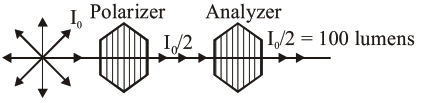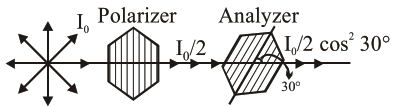# An unpolarized light`
Question:

An unpolarized light beam is incident on the polarizer of a polarization experiment and the intensity of light beam emerging from the analyzer is measured as 100 Lumens. Now, if the analyzer is rotated around the horizontal axis (direction of light) by $30^{\circ}$ in clockwise direction, the intensity of emerging light will be Lumens.

Solution:Assuming initially axis of Polarizer and Analyzer are parallelNow emerging intensity $=\frac{\mathrm{I}_{0}}{2} \cos ^{2} 30^{\circ}$

$=100\left(\frac{\sqrt{3}}{2}\right)^{2}=100 \times \frac{3}{4}=75$

Ans. 75